Ex 4.5

Chapter 4 Class 12 Determinants
Serial order wise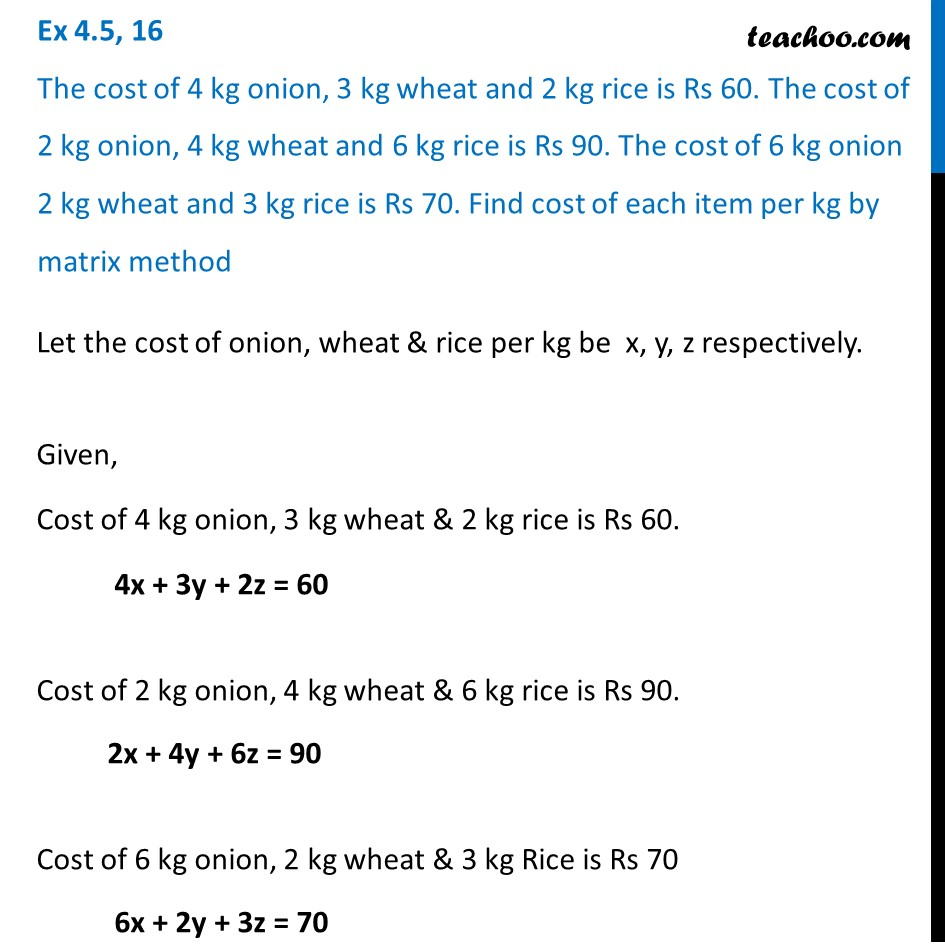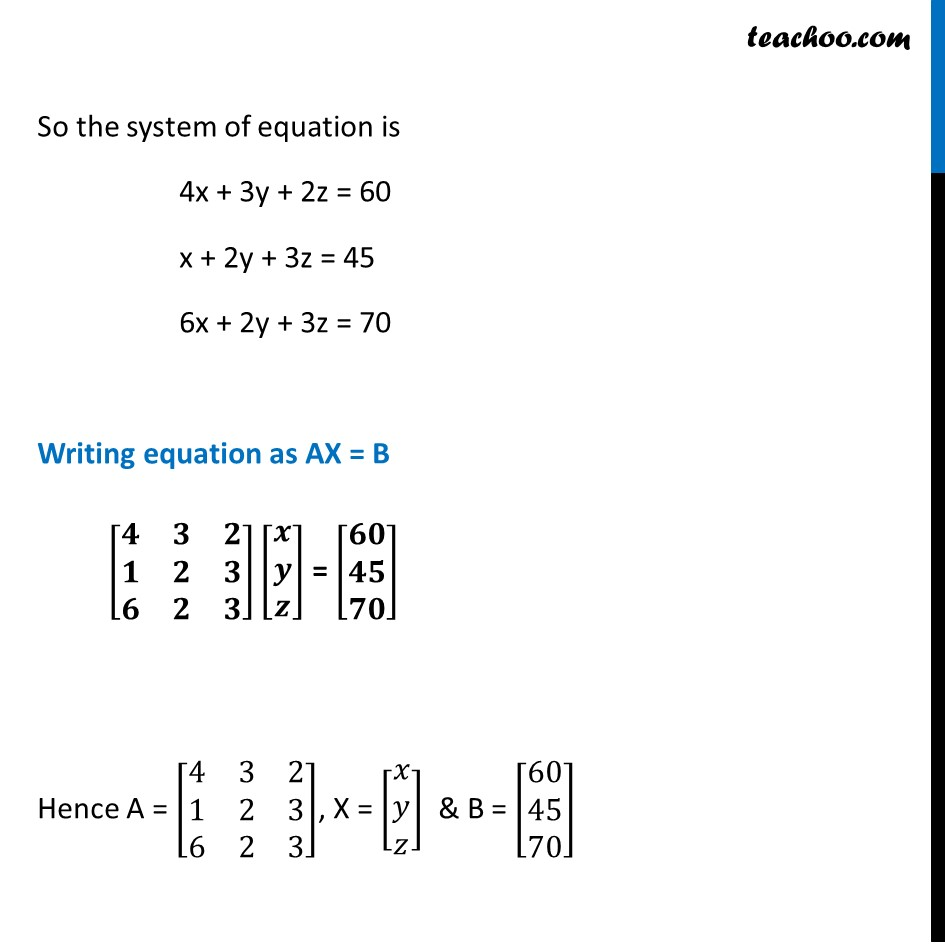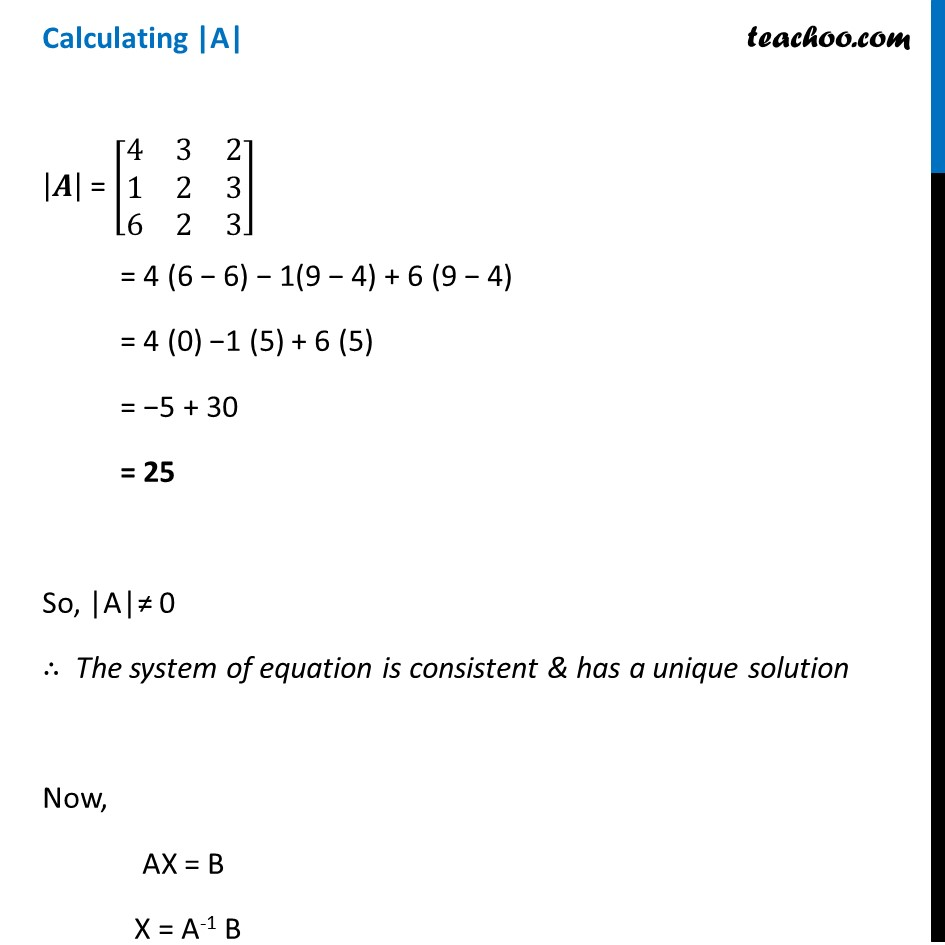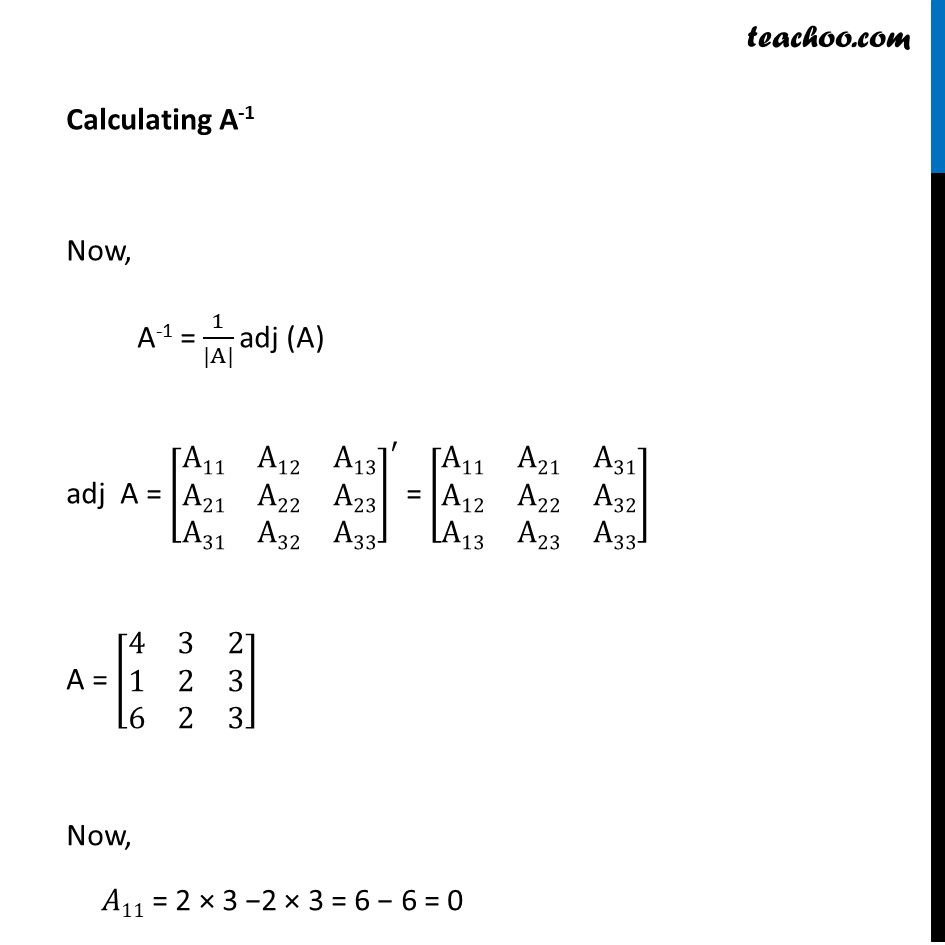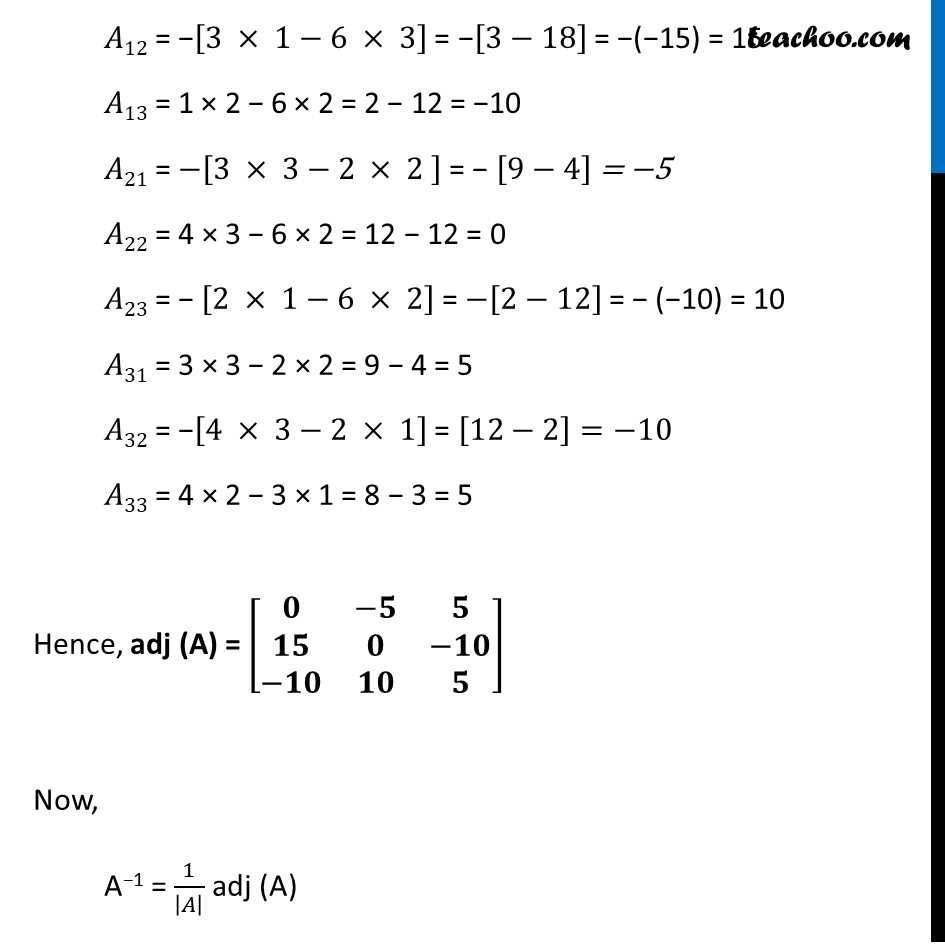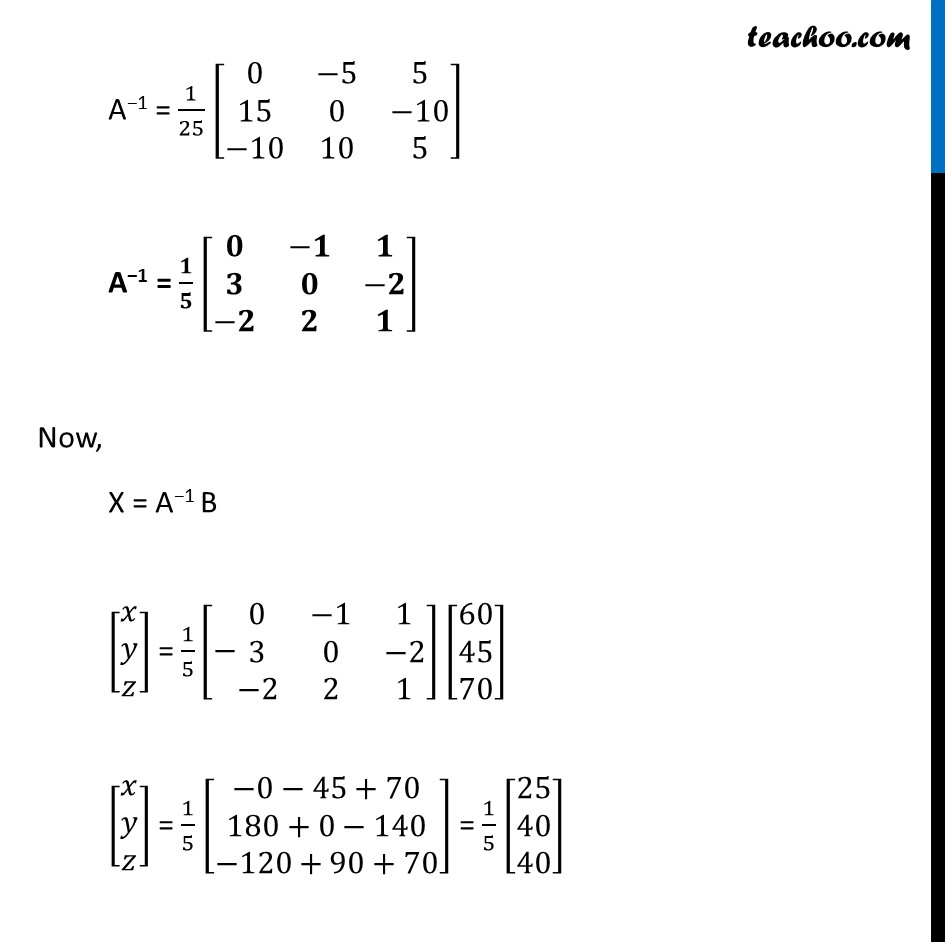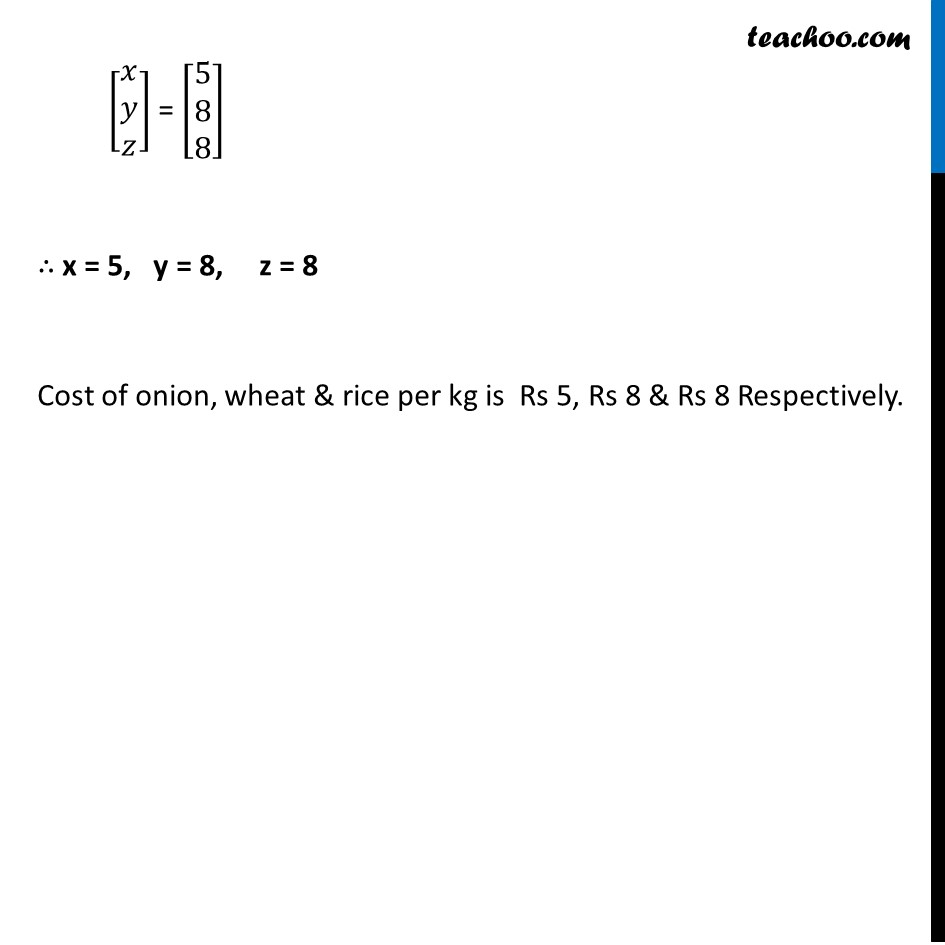Learn in your speed, with individual attention - Teachoo Maths 1-on-1 Class

### Transcript

Ex 4.5, 16 The cost of 4 kg onion, 3 kg wheat and 2 kg rice is Rs 60. The cost of 2 kg onion, 4 kg wheat and 6 kg rice is Rs 90. The cost of 6 kg onion 2 kg wheat and 3 kg rice is Rs 70. Find cost of each item per kg by matrix methodLet the cost of onion, wheat & rice per kg be x, y, z respectively. Given, Cost of 4 kg onion, 3 kg wheat & 2 kg rice is Rs 60. 4x + 3y + 2z = 60 Cost of 2 kg onion, 4 kg wheat & 6 kg rice is Rs 90. 2x + 4y + 6z = 90 Cost of 6 kg onion, 2 kg wheat & 3 kg Rice is Rs 70 6x + 2y + 3z = 70 So the system of equation is 4x + 3y + 2z = 60 x + 2y + 3z = 45 6x + 2y + 3z = 70 Writing equation as AX = B [■8(𝟒&𝟑&𝟐@𝟏&𝟐&𝟑@𝟔&𝟐&𝟑)][■8(𝒙@𝒚@𝒛)] = [■8(𝟔𝟎@𝟒𝟓@𝟕𝟎)] Hence A = [■8(4&3&2@1&2&3@6&2&3)], X = [■8(𝑥@𝑦@𝑧)] & B = [■8(60@45@70)] Calculating |A| |𝑨| = [■8(4&3&2@1&2&3@6&2&3)] = 4 (6 − 6) − 1(9 − 4) + 6 (9 − 4) = 4 (0) −1 (5) + 6 (5) = −5 + 30 = 25 So, |A|≠ 0 ∴ The system of equation is consistent & has a unique solution Now, AX = B X = A-1 B Calculating A-1 Now, A-1 = 1/(|A|) adj (A) adj A = [■8(A11&A12&A13@A21&A22&A23@A31&A32&A33)]^′ = [■8(A11&A21&A31@A12&A22&A32@A13&A23&A33)] A = [■8(4&3&2@1&2&3@6&2&3)] Now, 𝐴11 = 2 × 3 −2 × 3 = 6 − 6 = 0 𝐴12 = −[3 × 1−6 × 3] = −[3−18] = −(−15) = 15 𝐴13 = 1 × 2 − 6 × 2 = 2 − 12 = −10 𝐴21 = −[3 × 3−2 × 2 ] = − [9−4] = −5 𝐴22 = 4 × 3 − 6 × 2 = 12 − 12 = 0 𝐴23 = − [2 × 1−6 × 2] = −[2−12] = − (−10) = 10 𝐴31 = 3 × 3 − 2 × 2 = 9 − 4 = 5 𝐴32 = −[4 × 3−2 × 1] = [12−2]=−10 𝐴33 = 4 × 2 − 3 × 1 = 8 − 3 = 5 Hence, adj (A) = [■8(𝟎&−𝟓&𝟓@𝟏𝟓&𝟎&−𝟏𝟎@−𝟏𝟎&𝟏𝟎&𝟓)] Now, A−1 = 1/|𝐴| adj (A) A−1 = 1/25 [■8(0&−5&5@15&0&−10@−10&10&5)] A−1 = 𝟏/𝟓 [■8(𝟎&−𝟏&𝟏@𝟑&𝟎&−𝟐@−𝟐&𝟐&𝟏)] Now, X = A−1 B [■8(𝑥@𝑦@𝑧)] = 1/5 [−■8(0&−1&1@3&0&−2@−2&2&1)] [■8(60@45@70)] [■8(𝑥@𝑦@𝑧)] = 1/5 [█(−0−45+70@180+0−140@−120+90+70)] = 1/5 [■8(25@40@40)] [■8(𝑥@𝑦@𝑧)] = [■8(5@8@8)] ∴ x = 5, y = 8, z = 8 Cost of onion, wheat & rice per kg is Rs 5, Rs 8 & Rs 8 Respectively.